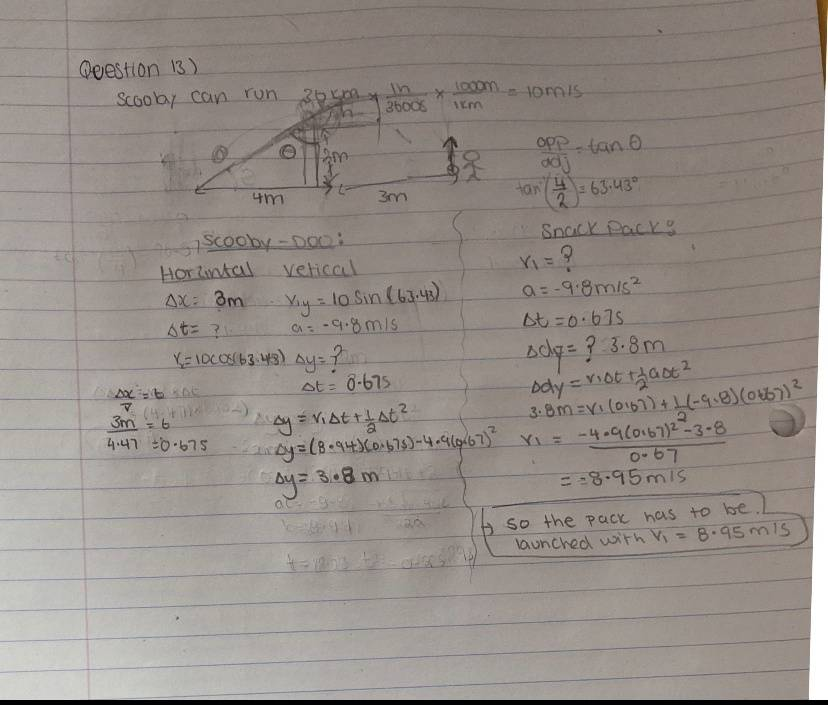# Mechanics; grade 12 Projectile motion

Summary:: Scooby-Doo wants a Scooby Snack. According to a website I looked up last night, Scooby can run at a speed of 36 km/h. He runs up a ramp (as shown, 2.0 meters high, 4.0 meters long) at that speed and off the edge. Three meters from the end of the ramp, Shaggy has a Scooby-Snack launcher which will fire a snack straight up. At the instant Scooby leaves the ramp, Shaggy fires the snack. What is the initial speed of the launched snack for Scooby to catch it in midair?Not sure if this correct. How do you find the X and Y components with no given angle, so I tried to find the angle of the hill.

[Moderator's note: Moved from a technical forum and thus no template.]

Last edited by a moderator:

PeroK
Homework Helper
Gold Member
2020 Award
How about ignoring gravity, as it is the same for Scooby and for the snack?

And, instead of calculating ##\theta##, why not take ##u_x = 2u_y##?

Lnewqban
Gold Member
Welcome, ttt6683!Your calculated angle for the ramp seems to be incorrect.
Therefore, your calculated values of ##V_x## and of ##V_y## for Scooby-Doo at the edge of the ramp are not correct either.

Last edited:
•PeroK
PeroK
Homework Helper
Gold Member
2020 Award
Welcome, ttt6683!Your calculated angle for the ramp seems to be incorrect.
Therefore, your calculated values of ##V_x## and of ##V_y## for Scooby-Doo at the edge of the ramp are not correct either.
Which is ironic, given there was no need to calcuate the angle!

Moral: avoid unnecessary calculations.

PeroK
Homework Helper
Gold Member
2020 Award
Not sure if this correct. How do you find the X and Y components with no given angle, so I tried to find the angle of the hill.

A neat way to do this is to observe that $$\frac {u_y}{u_x} = \tan \theta = \frac 2 4$$, hence $$u_x = 2u_y$$
And:
$$u^2 = u_x^2 + u_y^2 = 5u_y^2$$ hence $$u_y = \frac u {\sqrt{5}} \ \ \text{and} \ \ u_x = \frac{2u}{\sqrt{5}}$$
Where ##u = 10m/s## is the initial launch speed.